nextnano.com  nextnano³  Download | Search | Copyright | Publications  * password protected nextnano³ software2D GaN nanowire

# nextnano3 - Tutorial

## Energy dispersion of a cylindrically shaped GaN nanowire

Author: Stefan Birner

If you want to obtain the input files that are used within this tutorial, please check if you can find them in the installation directory.
If you cannot find them, please submit a Support Ticket.
` -> 2DGaN_nanowire_nn3.in / *_nnp.in - `input file for the nextnano3 and nextnano++ software```  ```

## Energy dispersion of a cylindrically shaped GaN nanowire

In this tutorial we study the electron and hole energy levels of a two-dimensional freestanding GaN nanowire of cylindrical shape.

The tutorial is based on the following paper:

X.W. Zhang, J.B. Xia
Optical properties of GaN wurtzite quantum wires
J. Phys.: Condens. Matter 18, 3107 (2006)

• We assume a cylindrically shaped GaN nanowire (wurtzite structure) that has a radius of 2 nm with infinite barriers so that the wave functions are zero at the nanowire boundary. This assumption is consistent to the above cited paper. The GaN nanowire is shown in red in the following figure. The GaN nanowire is discretized on a mesh with a grid resolution of 0.05 nm.### Electrons

• The following figure shows the electron states as a function of k of the GaN nanowire. It is in excellent agreement with Fig. 1 of the above cited paper. All states are two-fold degenerate due to spin. In addition, the 2nd and 3rd state are degenerate, as well as the 4th and the 5th.
The ground state has quantum number L = 0. For L /= 0, the states are degenerate due to L = +/`-` 1.
The energy levels increase with increasing k as quadratic terms of k (parabolic dispersion).

Technical details: We calculated the electron energy levels at kz = 0 with nextnano³ numerically by solving the 2D single-band Schrödinger equation.
The parabolic dispersion for kz /= 0 has been calculated analytically using Ei(kz)=Ei + hbar2 kz2 / (2m*), i.e. not with nextnano³.
The eigenvalues for kz = 0 can be found in the following file:``` Schroedinger_1band/ev_cb1_sg1_deg1.dat```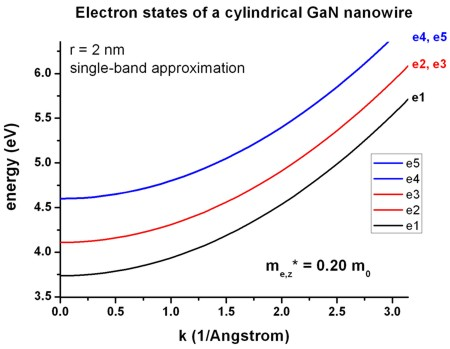• The wave function (psi²) of the electron ground state at k = 0 is shown in this figure.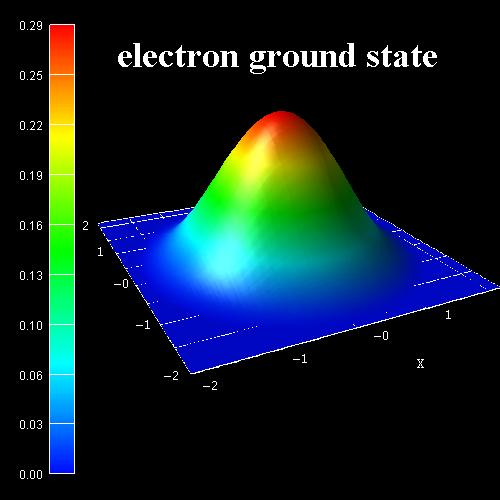### Holes

• The following figures show the ground state wave function (psi²) of the hole (left figure) and the 1st excited hole state (right figure) as calculated within the 6-band k.p approximation at k = 0.
According to the above cited paper, the right figure would be the ground state for GaN nanowires with a radius < 0.7 nm. Because our nanowire has a radius of 2 nm, the ground state wave function is according to the left figure.
Following the paper of Zhang and Xia, this means that the probability for electron-hole transitions (e1-h1) is not very high at a radius of 2 nm because the wave functions don't have much overlap and the electron ground state has L = 0, whereas the hole ground state has L = +/`-` 1 (dark exciton effect).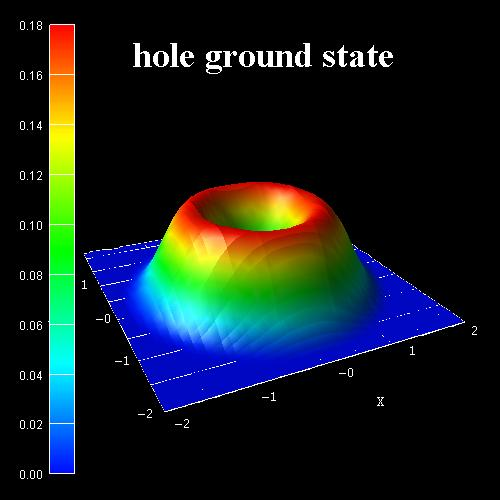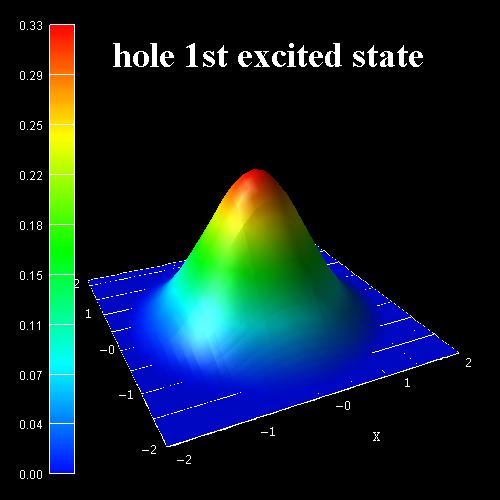• The following figure shows the hole states as a function of k of the GaN nanowire as calculated with 6-band k.p theory. It corresponds to Fig. 2 and Fig. 3 of the paper of Zhang and Xia. Note that the authors assumed the hole energies to be positive.
All states are two-fold degenerate, i.e. h1=h2, h3=h4, h5=h6, ...
The nextnano³ results are a bit different. Several reasons could explain this:
`- `The authors use the "cylindrical approximation" for the k.p parameters. However, the parameters that they are citing are not exactly cylindrical. Thus for our calculations, we had to employ the parameters that they were citing (without making use of the cylindrical approximation).
`- `Our cylinder does not have exactly cylindrical symmetry. It is approximated to be cylindrical by a rectangular grid with a grid resolution of 0.05 nm.
`- `For the k.p parameters that are given in the paper it must hold:```   A5 = 1/2 (L1 - M1) ```must be equal to```   A5 = 1/2 N1   ```but they differ by` 0.0064`.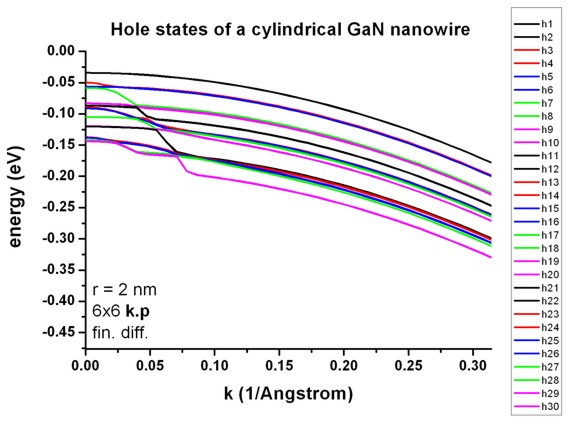The data that has been plotted in this figure is contained in this file:
`Schroedinger_kp/kpar2D_disp_0_1_hl_6x6kp_ev_min001_ev_max030.dat`

In the input file, one can specify the number of k|| = kz points.

``` \$quantum-model-holes``````  ...  num-kp-parallel = 41 ```
Note:` 41 `points means 16 minutes CPU time (Intel i5, 2015). If one uses only` 1`, then one only calculates the k.p states at kz=0 and the calculation takes less than a minute.

• Zhang and Xia used the following 6-band k.p parameters:

Crystal field and spin-orbit splitting energies:
```  Deltacr = 0.021   Deltaso = 0.018```

"Dresselhaus" parameters:

``` Zhang/Xia           nextnano³  ==========        =============  L = 6.3055  <==>  L1 = -6.3055 - 1 = -7.3055   (!) ==> ```The definition of the k.p Hamiltonians differs.```  M = 0.1956  <==>  M1 = -0.1956 - 1 = -1.1956   (!) ==> ```The definition of the k.p Hamiltonians differs.```  N = 0.3813  <==>  M2 = -0.3813 - 1 = -1.3813   (!) ==> ```The definition of the k.p Hamiltonians differs.```  R = 6.1227  <==>  N1 = -6.1227  S = 0.4335  <==>  M3 = -0.4335 - 1 = -1.4335   (!) ==> ```The definition of the k.p Hamiltonians differs.```  T = 7.3308  <==>  L2 = -7.3308 - 1 = -8.3308   (!) ==> ```The definition of the k.p Hamiltonians differs.```  Q = 4.0200  <==>  N2 = -4.0200 ```
Conversion to "Luttinger" parameters:

``` A1 = L2 + 1  = -8.3308 + 1      = -7.3308       (!) ==> ```The definition of the k.p Hamiltonians differs.```  A2 = M3 + 1  = -1.4335 + 1      = -0.4335       (!) ==> ```The definition of the k.p Hamiltonians differs.```  A3 = M2 - L2 = -0.3813 + 7.3308 =  6.9495  A4 = 1/2 (L1 + M1 - 2M3)        = -2.81705  A5 = 1/2 (L1 - M1)              = -3.05495      (!) ==> ```inconsistent to``` -3.06135     = 1/2  N1                    = -3.06135      (!) ==> ```inconsistent to``` -3.05495  A6 = SQRT(2)/2 N2               = -2.84256926 ```

• cylindrical (axial) approximation
```       L           -  M           - R   = 0   ```(Zhang)```       L1          -  M1          - N1  = 0   ```(nextnano³)```  ==> (A2+A4+A5-1) - (A2+A4-A5-1) - 2A5 = 0       A1 - A2   = -A3 = 2 A4       A3 + 4 A5 =       SQRT(2) A6       Delta2 = Delta3 = 1/3 Deltaso  ```